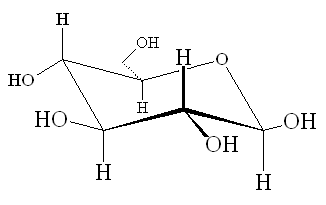# Question #6e583

Aug 17, 2016

Here's what I get.

#### Explanation:

The structure of glucose isElectron domain geometries

Every carbon atom is directly attached to four other atoms (steric number = 4). Its electron domain geometry is therefore tetrahedral.

Every oxygen atom has two lone pairs and is directly attached to two other atoms (steric number = 4). Its electron domain geometry is therefore tetrahedral.

Molecular geometries

Every carbon atom is directly attached to four other atoms. Its molecular geometry is therefore tetrahedral.

Every oxygen atom has two lone pairs and two bonding pairs. Its molecular geometry is therefore bent.

Hybridization

Every carbon and oxygen atom has a steric number of 4. Their hybridization is therefore $s {p}^{3}$.

Bond angles

All bond angles are approximately equal to the tetrahedral bond angle of 109.5°.